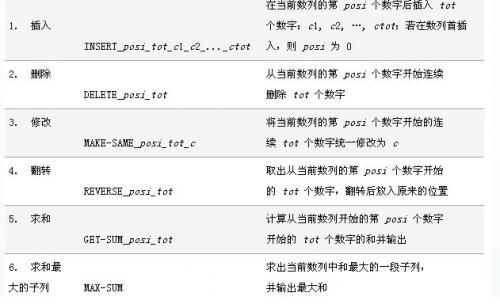# 题目大意# 题目大意

$T$中可能存在?通配符，可以匹配任何英文字符。

# 题目大意

$F_i={j<i}\frac{q_iq_j}{(i-j)^2}-{j>i}\frac{q_iq_j}{(i-j)^2}$

$$E_i=\frac{F_i}{q_i}​$$

# 题目大意

• $1\ a\ b\ c$ 在第$a$个位置到第$b$个位置，每个位置加入一个数$c$。
• $2\ a\ b\ c$ 询问从第$a$个位置到第$b$个位置，第$c$大的数是多少。
###### Your browser is out-of-date!

Update your browser to view this website correctly. Update my browser now

×